# 深度学习-初识CNN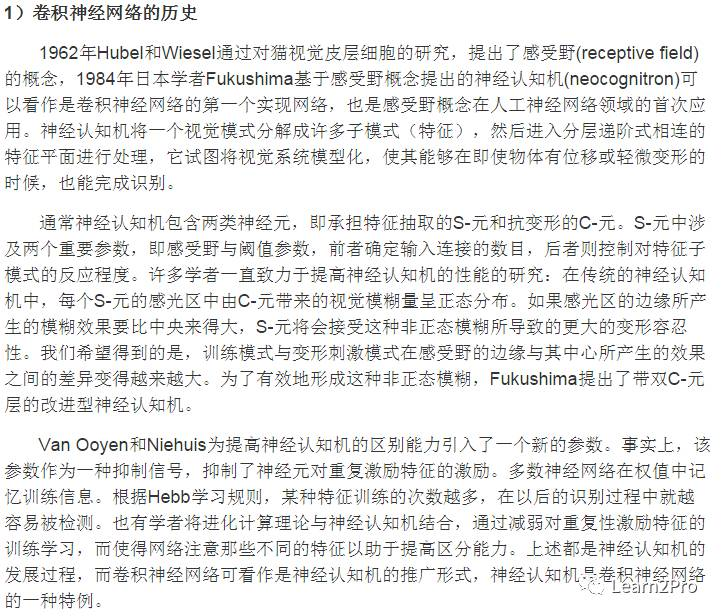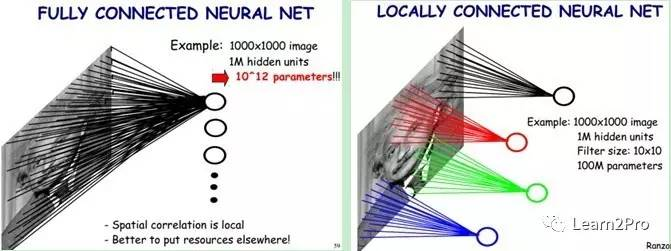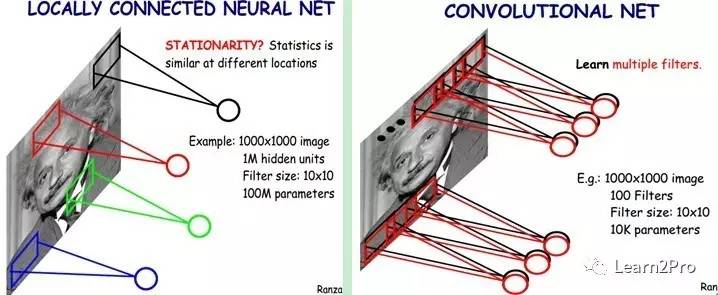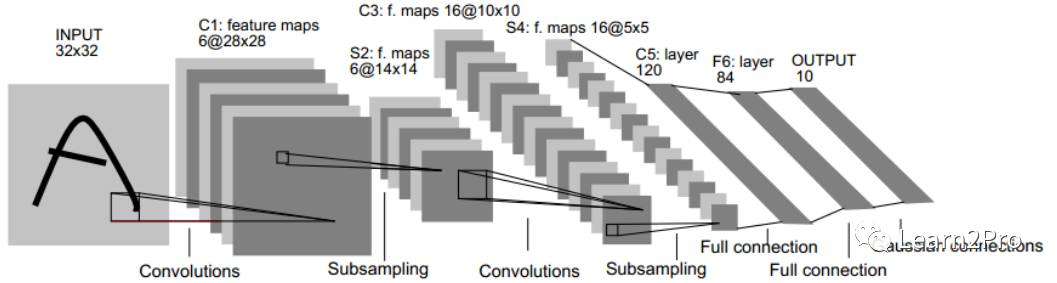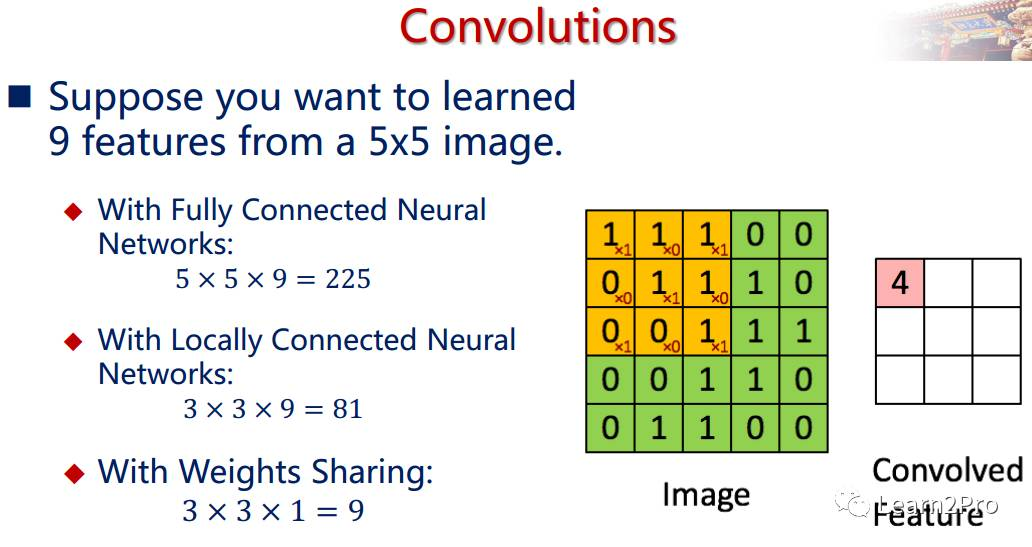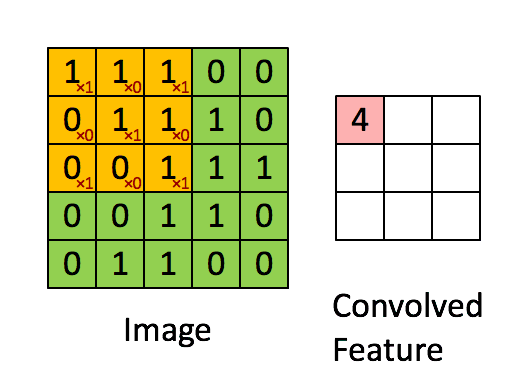Max-Pooling: 选择Pooling窗口中的最大值作为采样值；

Mean-Pooling: 将Pooling窗口中的所有值相加取平均，以平均值作为采样值；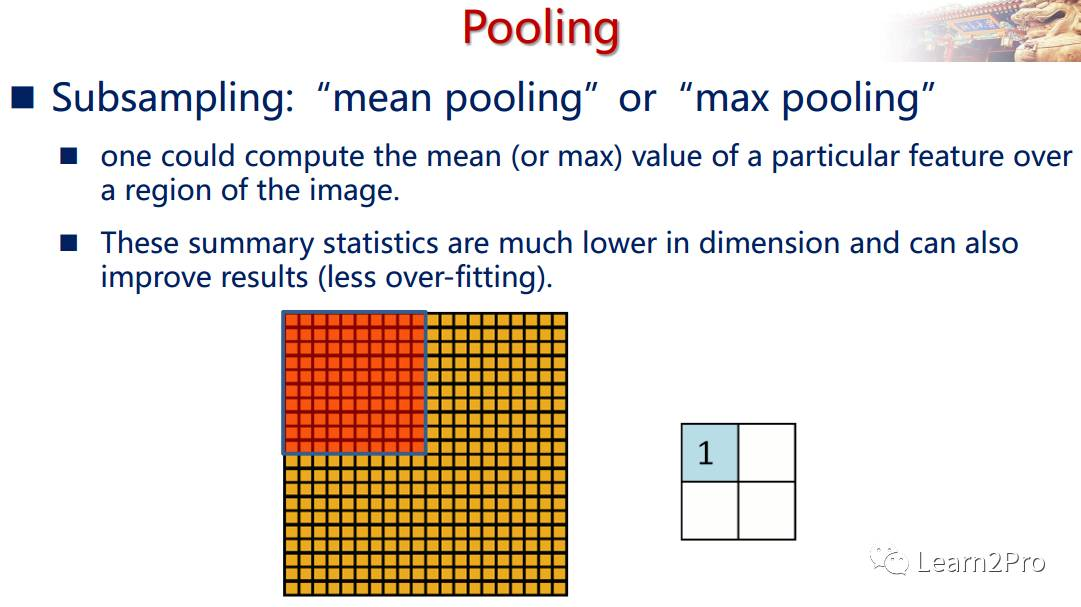LeNet-5网络详解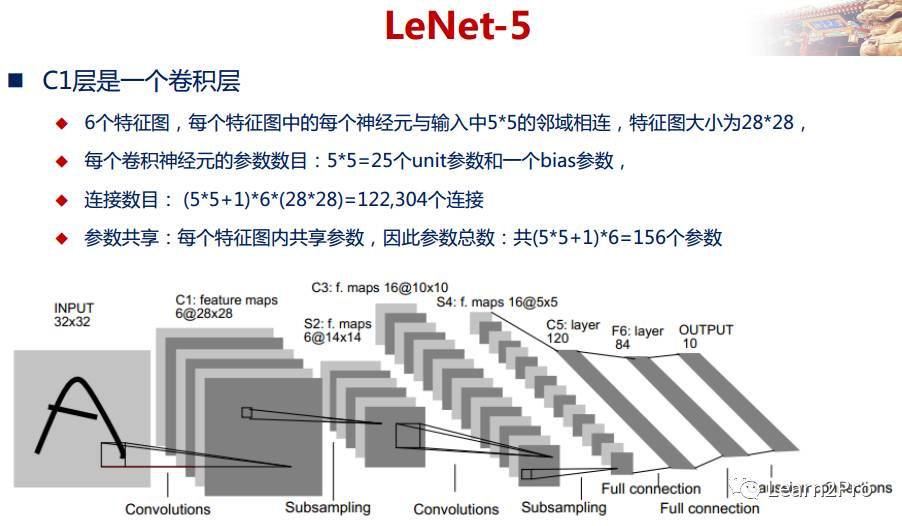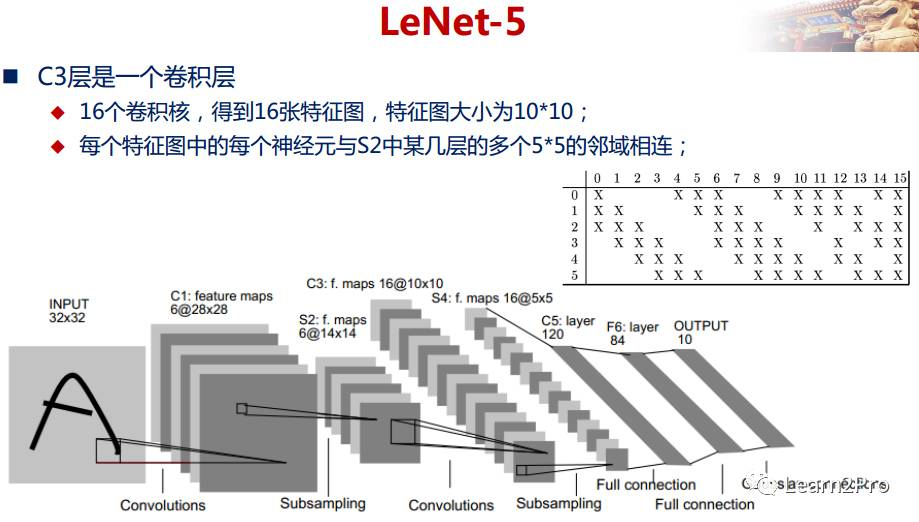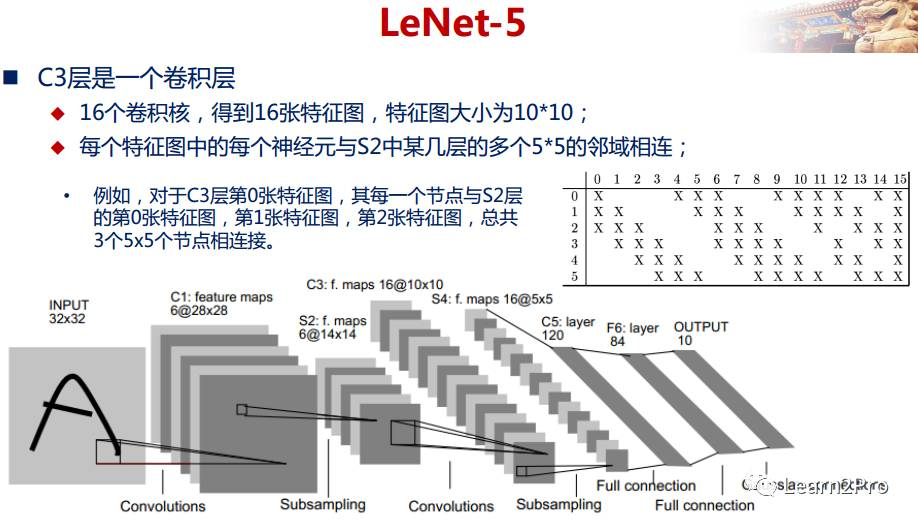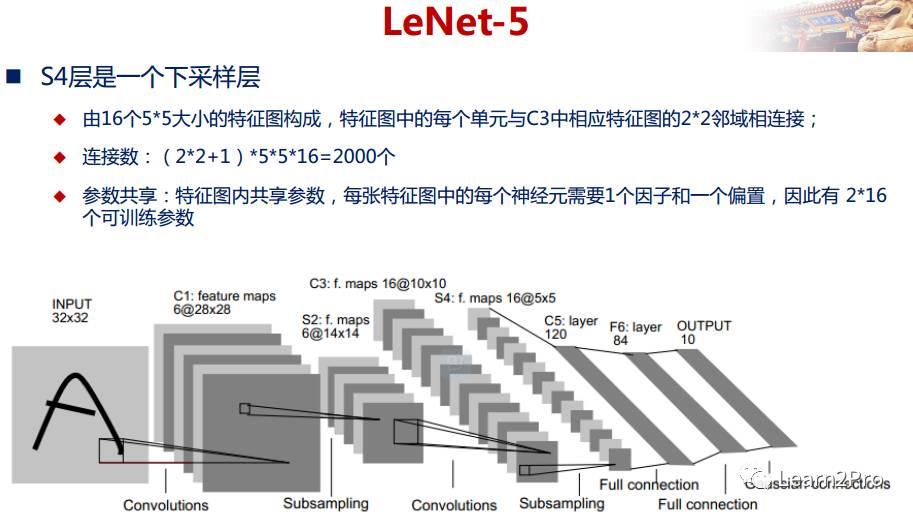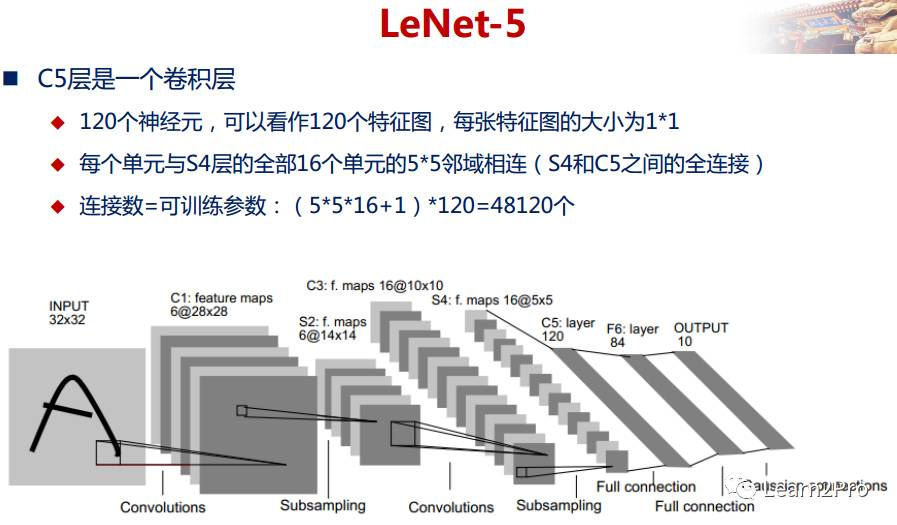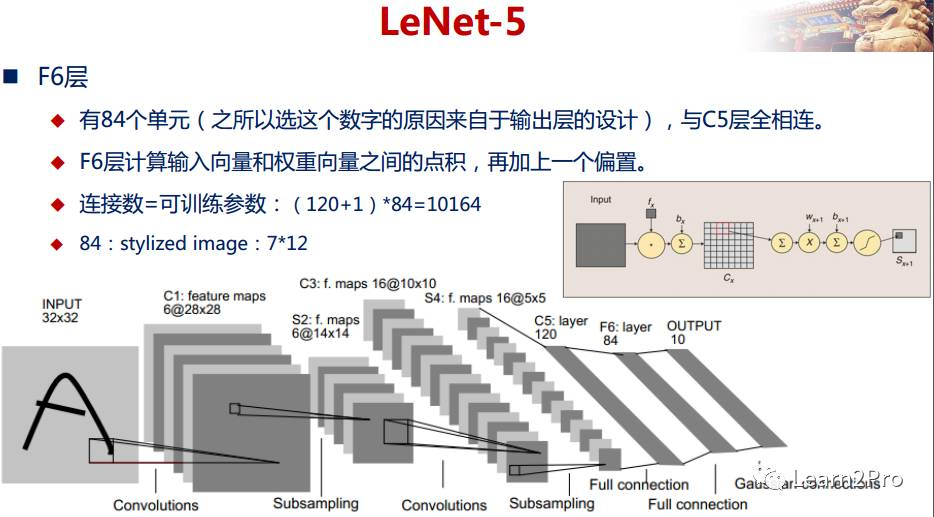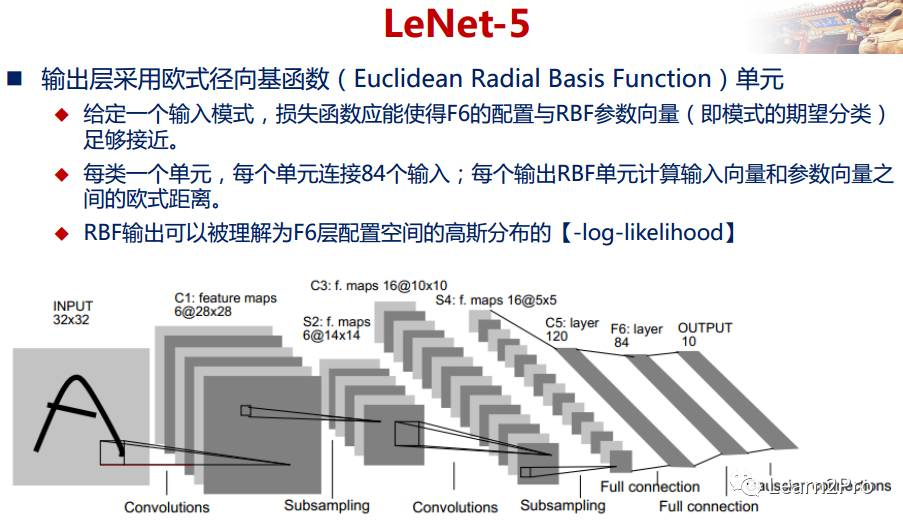LeNet-5网络在MNIST数据集上的结果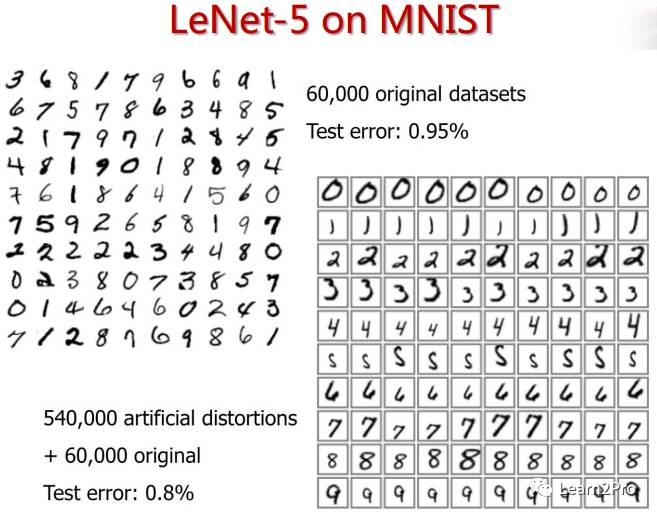Flabby-bird整个的学习过程如下：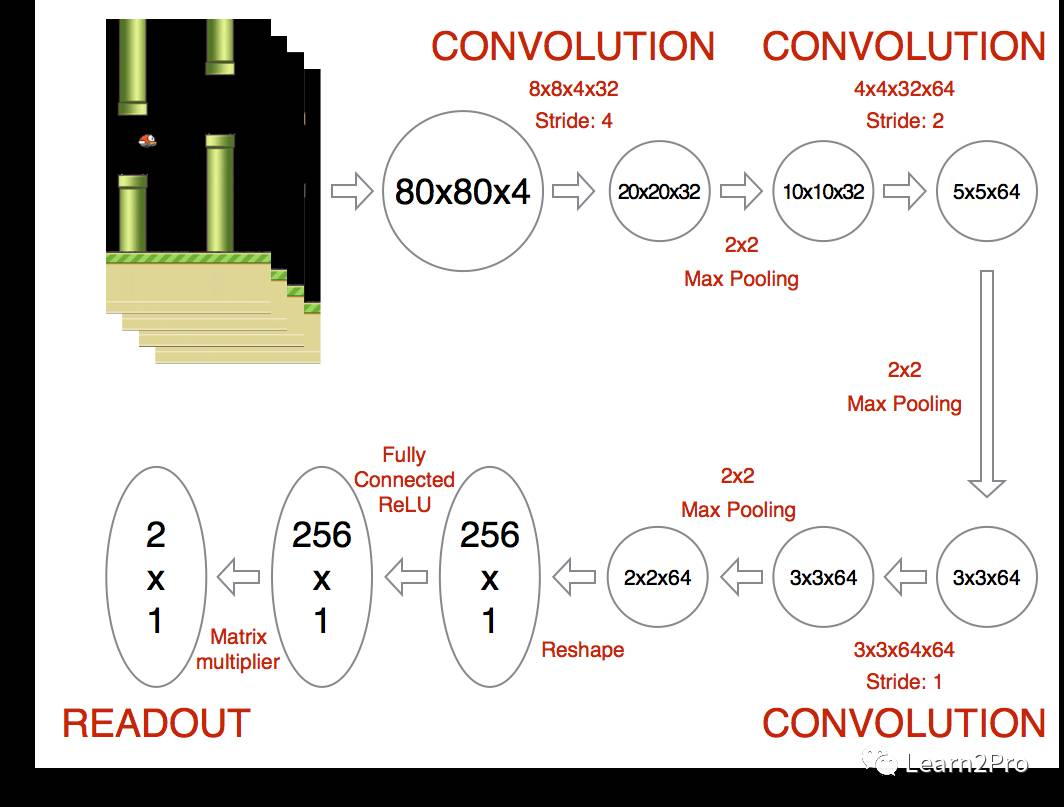Initialize replay memory D to size N
Initialize action-value function Q with random weights
for episode = 1, M do
Initialize state s_1
for t = 1, T do
With probability ϵ select random action a_t
otherwise select a_t=max_a Q(s_t,a; θi)
Execute action a_t in emulator and observe r_t and s
(t+1)
Store transition (s_t,a_t,r_t,s_(t+1)) in D
Sample a minibatch of transitions (s_j,a_j,r_j,s_(j+1)) from D
Set y_j:=
r_j for terminal s_(j+1)
r_j+γ*max_(a^’ ) Q(s_(j+1),a’; θi) for non-terminal s(j+1)
Perform a gradient step on (y_j-Q(s_j,a_j; θ_i))^2 with respect to θ
end for
end for